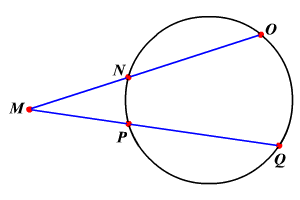# Intersecting Secants Theorem

If two secant segments are drawn to a circle from an exterior point, then the product of the measures of one secant segment and its external secant segment is equal to the product of the measures of the other secant segment and its external secant segment.In the circle, $\stackrel{¯}{\mathit{\text{M}}\mathit{\text{O}}}$ and $\stackrel{¯}{\mathit{\text{M}}\mathit{\text{Q}}}$ are secants that intersect at point $\mathit{\text{M}}$ .

So, $\mathit{\text{M}}\mathit{\text{N}}\cdot \mathit{\text{M}}\mathit{\text{O}}=\mathit{\text{M}}\mathit{\text{P}}\cdot \mathit{\text{M}}\mathit{\text{Q}}$ .

Example :

In the circle shown, if $\mathit{\text{M}}\mathit{\text{N}}=10,\mathit{\text{N}}\mathit{\text{O}}=17,\mathit{\text{M}}\mathit{\text{P}}=9$ , then find the length of $\mathit{\text{P}}\mathit{\text{Q}}$ .

Substitute.

$\begin{array}{l}\mathit{\text{M}}\mathit{\text{N}}\cdot \mathit{\text{M}}\mathit{\text{O}}=\mathit{\text{M}}\mathit{\text{P}}\cdot \mathit{\text{M}}\mathit{\text{Q}}\\ \left(\mathit{\text{M}}\mathit{\text{N}}\right)\left(\mathit{\text{M}}\mathit{\text{N}}+\mathit{\text{N}}\mathit{\text{O}}\right)=\left(\mathit{\text{M}}\mathit{\text{P}}\right)\left(\mathit{\text{M}}\mathit{\text{P}}+\mathit{\text{P}}\mathit{\text{Q}}\right)\\ \left(10\right)\left(10+17\right)=\left(9\right)\left(9+\mathit{\text{P}}\mathit{\text{Q}}\right)\\ \left(10\right)\left(27\right)=\left(9\right)\left(9+\mathit{\text{P}}\mathit{\text{Q}}\right)\\ 270=81+9\mathit{\text{P}}\mathit{\text{Q}}\\ 189=9\mathit{\text{P}}\mathit{\text{Q}}\\ 21=\mathit{\text{P}}\mathit{\text{Q}}\end{array}$

Therefore, $\mathit{\text{P}}\mathit{\text{Q}}=21$ units.